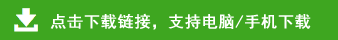# 二年级数学下册各单元测试卷

一、口算我最快。（8分）

72+8= 45－9=  36+6=  32－8=   18÷3=

8×7=  30÷6=  43－4=   54－30=  30÷5=

6×5=  25÷5=  5×5=  49+7=   15÷5=

6×6=  72－7=   10÷5=   4×5=   20－8=

24÷4=   4×6=  36÷6=   20÷5=   32+9=

16÷4=   18+8=   4×5=  4×2=  90－4=

2×3=  12÷3=   28+7=  2×2=  6×3=

45÷5=   12÷2=  68+4=  26+50=   90－9=

1、（）×5=15   6×（）=243×（）=18

（）×4=20  五（）三十   （）四十二

（）六十二  五（）二十五 （）四得八